# Stochastic Modeling

It is often very useful to have in mind a simple conceptual model for the variabilty you observe in a time series. Stochastic process provide such convenient conceptual model. That is, we compare the observed variability with what can be generated by, essentially, some kind of random number generator. This is particular useful in assessing the statistical significance of any structure we might see in the data. The stochastic process becomes what we call the null hypothesis---that is, the hypothesis that nothing particularly interesting is going on. When we see structure in the data that is very different from that which occurs in the stochastic process, then reject the null hypothesis, meaning that there are grounds for believing something interesting is happening in the data. This can all be done in a statistically formal sense, but it is also very useful to do informally, as we will do here.
The trick with stochastic processes is finding a suitable choice of process and appropriate parameter values. Here you will get to know a very simple but flexible stochastic process called damped fractional Brownian motion. All the tools you need to work with this process are in jLab. In this demo, you will get a functional appreciation of how to work with this type of process. All the gory details of the theory can be found here.

# Topics Covered

1. Generating white noise with Matlab's randn
2. Generating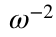red noise by applying Matlab's cumsum to white noise
3. Generating a hybrid process, transitioning from white to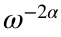, using the jLab routine maternoise
4. Understanding the relation of this hybrid process to other well-known processes

# White Noise

We will seek a stochastic process that is suitable for comparison with the m1224 velocity data. The simplest kind of stochastic process is discrete Gaussian white noise, which we make using Matlab's randn function.
use m1244
cv=cv(:,4);%Use the fourth (deepest) depth, as before
N=length(num);
cveps=randn(N,1)+1i*randn(N,1);
cveps=cveps.*std(cv)./std(cveps); %Set standard deviation
Here we have made a sequence of complex-valued noise, with independent realizations in the real and imaginary components, having the same length as the data. Also, we have set the standard deviation of the noise to be the same as that of the observed time series. The ‘eps’ stands for epsilon, a symbol often used to designate a noise process.
figure,
subplot(2,1,1),uvplot(yearfrac(num),cv),axis tight
subplot(2,1,2),uvplot(yearfrac(num),cveps+mean(real(cv))),axis tight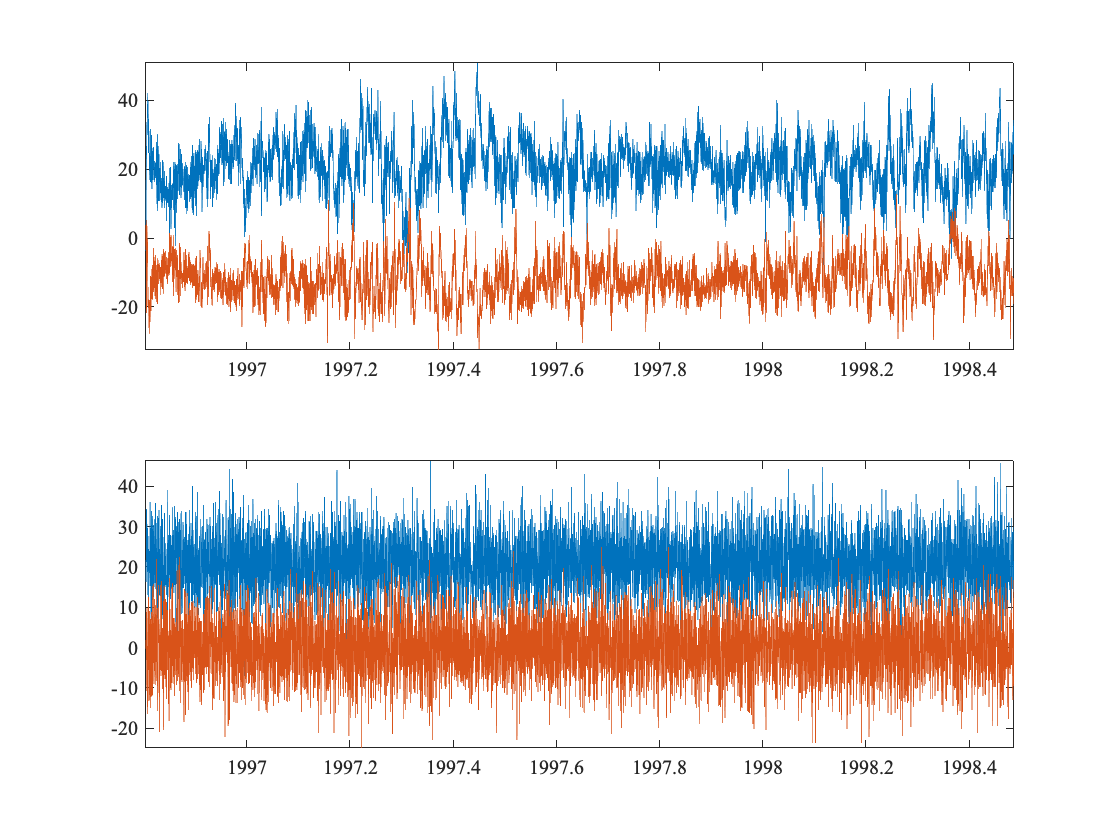For visual comparison, I'm adding in the mean of the real part in order to shift the blue curve of the noise. It is clear that this is not a good match, as the degree of roughness of the noise is too high compared the data. This is more clear in the spectral domain.
psi=sleptap(length(cv),16);
SLEPTAP calculating tapers of length 512.
SLEPTAP interpolating to length 7371.
[f,spp,snn]=mspec(2/24,cv-mean(cv),psi);
[f,sppeps,snneps]=mspec(2/24,cveps,psi);
clf,
plot(f/2/pi,[snn spp snneps sppeps]),xlim([f(2) f(end)]/2/pi),xlog,ylog,ylim(10.^[-1 3])
linestyle T U D k
title('Rotary Spectra of Signal vs. Noise')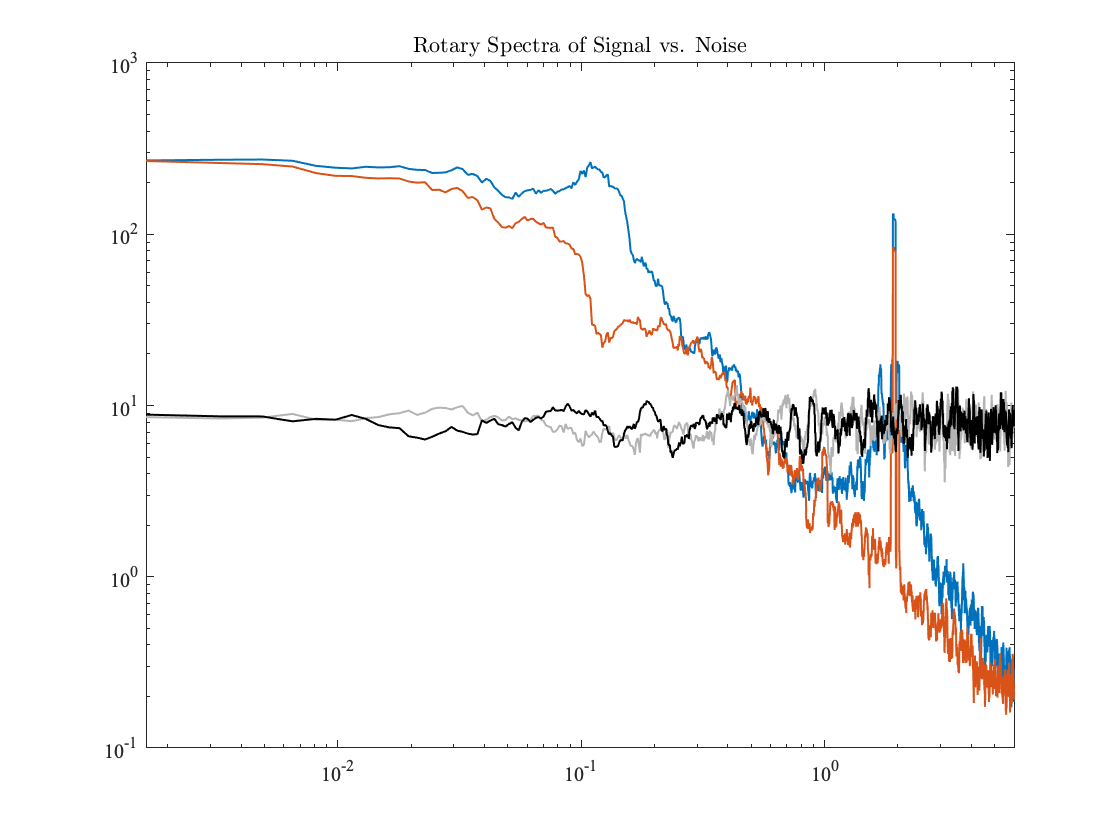The spectrum of the white noise, as you can see, is flat. This is why it is called white. That term is a reference to light. ‘White’ in this context means ‘having energy equally distributed across all frequencies,’ and therefore means that the spectrum is flat. Clearly this is a poor fit to the data. It has too much energy at high frequencies, and too little at low frequencies. This is apparent in the time series plot, but is much more clear in the spectrum.
Note that one thing which is working well is the representation of the low frequencies, as the data is also white or flat below about one cycle per 10 days. However, setting the noise variance to match the signal variance has led to the low-frequency spectral levels of the noise being far too low compared with the low-frequency spectral values of the signal.
The ‘noise’ portion of the name ‘Gaussian white noise’ is used because the process is unstructured, and therefore noiselike, as opposed to ‘signal’. The terms ‘signal’ literally means something which contains information, but in time series analysis it is used to refer to the structured portion of variability.
Finally the ‘Gaussian’ portion of the name refers to their probability distribution. If you make the histograms of a time series output by randn, you will find that it is indeed Gaussian. Recall that saying something has a Gaussian distribution is the same as saying that it has a normal distribution; this latter term apparently arose because Gaussian distributions are so common.

# Red Noise

The term ‘red noise’ has two meanings. Generally, it means a stochastic process that has more energ at lower frequencies that at higher frequencies, in other words, having a spectrum that slopes up toward zero frequency. This kind of noise is quite common. The opposite type of noise, blue noise, has more energy at high frequencies; this is more rarely encountered.
More specifically, the term ‘red noise’ is sometimes used to indicate noise having a spectrum proportional to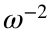. To be explicit, we will always refer to the value of the spectral slope.
Red noise with a -2 spectral slope can be generated very simply. As we saw in class from the differentiation theorem, differentiating in the time domain is equivalent to a mulitiplication in the frequency domain by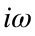. Let's say the orignal signal is a position or displacement, and its derivative is a velocity. If the original position signal has a -2 spectral slope, then the corresponding velocity has a slope of zero. This is because the spectrum is the magnitude squared of the Fourier transform, so that when we differentiate something, we mulitiply its spectrum by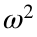.
Thus,red noise, when differentiated, leads to white noise. Working this backwards, integrating or cumululatively summing white noise should give usred noise. Here, we will subtract the mean after cumsumming, because otherwise the mean value can become very large.
cveps=cumsum(randn(N,1)+1i*randn(N,1));
cveps=cveps.*std(cv)./std(cveps);
cveps=cveps-mean(cveps); %Subtract the mean
figure,
subplot(2,1,1),uvplot(yearfrac(num),cv),axis tight
subplot(2,1,2),uvplot(yearfrac(num),cveps+mean(real(cv))),axis tight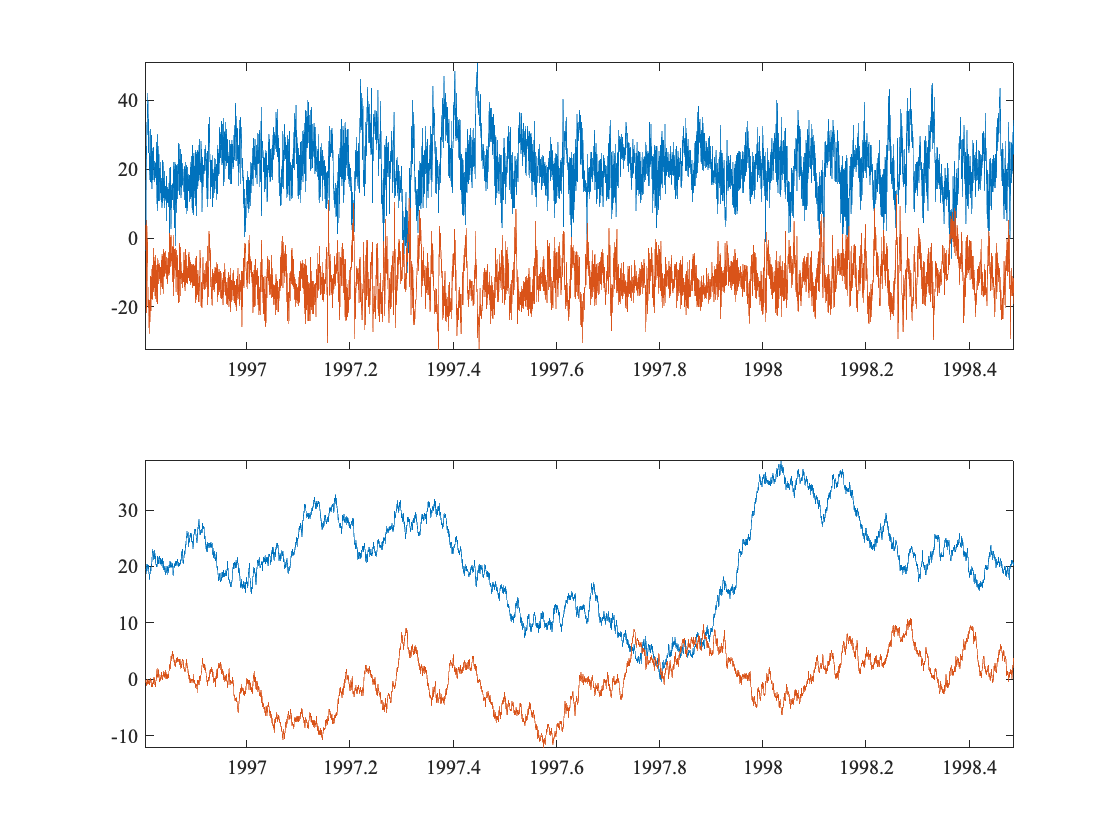Okay, well this doesn't look right either. Instead of being too rough, now the noise appears too smooth. Let's check out the spectrum.
%Note that this code is unchanged from above
[f,spp,snn]=mspec(2/24,cv-mean(cv),psi);
[f,sppeps,snneps]=mspec(2/24,cveps,psi);
clf,
plot(f/2/pi,[snn spp snneps sppeps]),xlim([f(2) f(end)]/2/pi),xlog,ylog,ylim(10.^[-1 3])
linestyle T U D k
title('Rotary Spectra of Signal vs. Noise')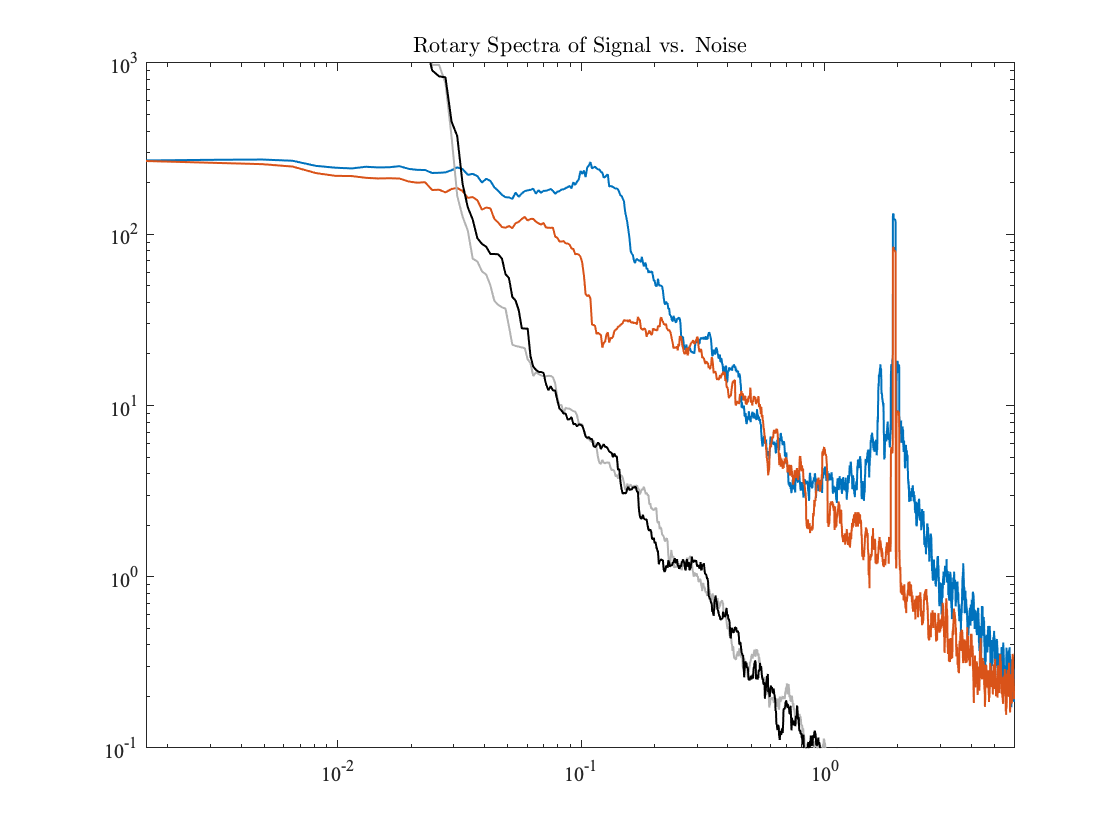Apparently, setting the variances to be the same was not the best idea if we want the spectra to be similar. Instead we would like the sloped parts to have about the same magnitude in the noise as in the data. It is possible to do this using an objective fit, such a least-squares fit over some range of frequencies, but to be honest the old-fashioned practice that we call ‘eyeballing it’---making it match according to visual inspection---is perfectly sufficient for most purposes.
cveps=cveps*5; %Take a guess
[f,sppeps,snneps]=mspec(2/24,cveps,psi);
clf,
plot(f/2/pi,[snn spp snneps sppeps]),xlim([f(2) f(end)]/2/pi),xlog,ylog,ylim(10.^[-1 3])
linestyle T U D k
title('Rotary Spectra of Signal vs. Noise')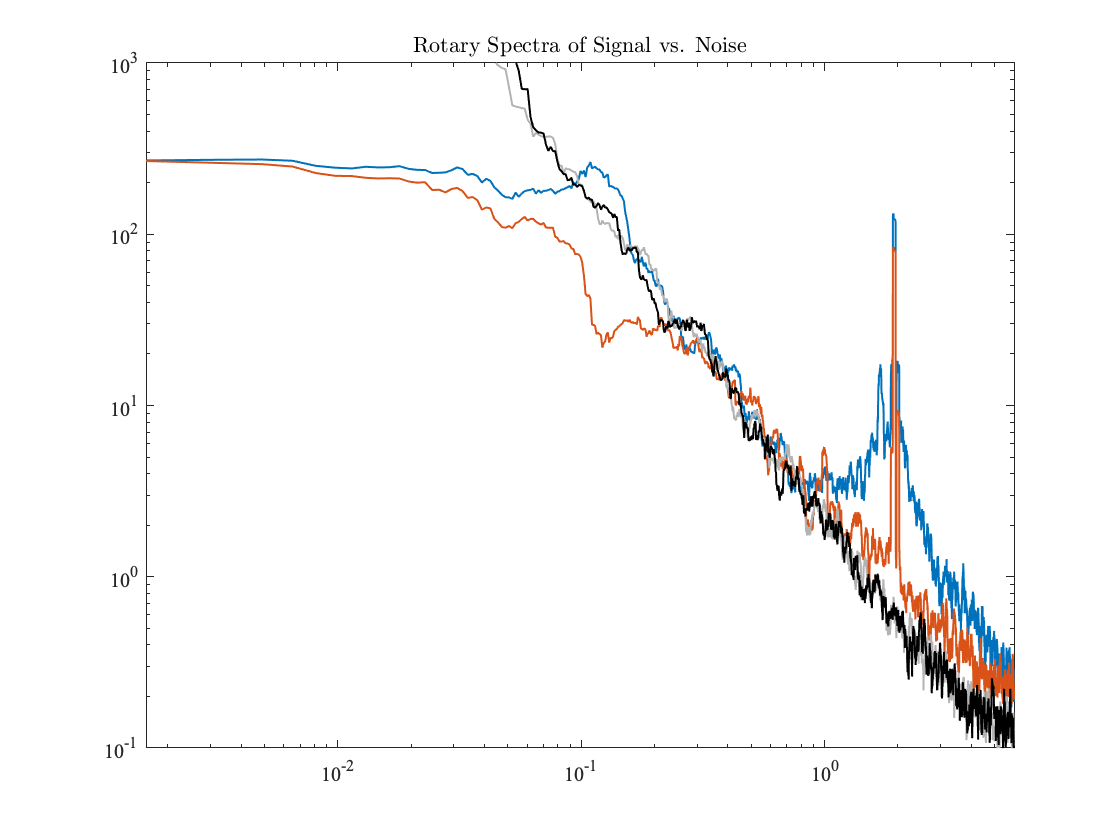Here what I have done is to completely take a guess. I just guessed that multiplying the noise by a factor of five would make the spectra fit better, with the intention of iterating that. However the first guess looks great. So now we can see thatred noise is a good fit to the sloped high-frequency portion of the spectrum. However, the low-frequency energy is now vastly too high. Moreover, the standard devation is an order of mangitude too high.
std(cv),std(cveps)
ans = 9.7245
ans = 48.6224
Moreover, now the time series have very different magnitudes. Note the different y-axis limits in these plots.
figure,
subplot(2,1,1),uvplot(yearfrac(num),cv),axis tight,ax=axis;
subplot(2,1,2),uvplot(yearfrac(num),cveps+mean(real(cv))),axis tight
hlines(ax(3:4),'k:') %The lines show the y-axis of the upper plot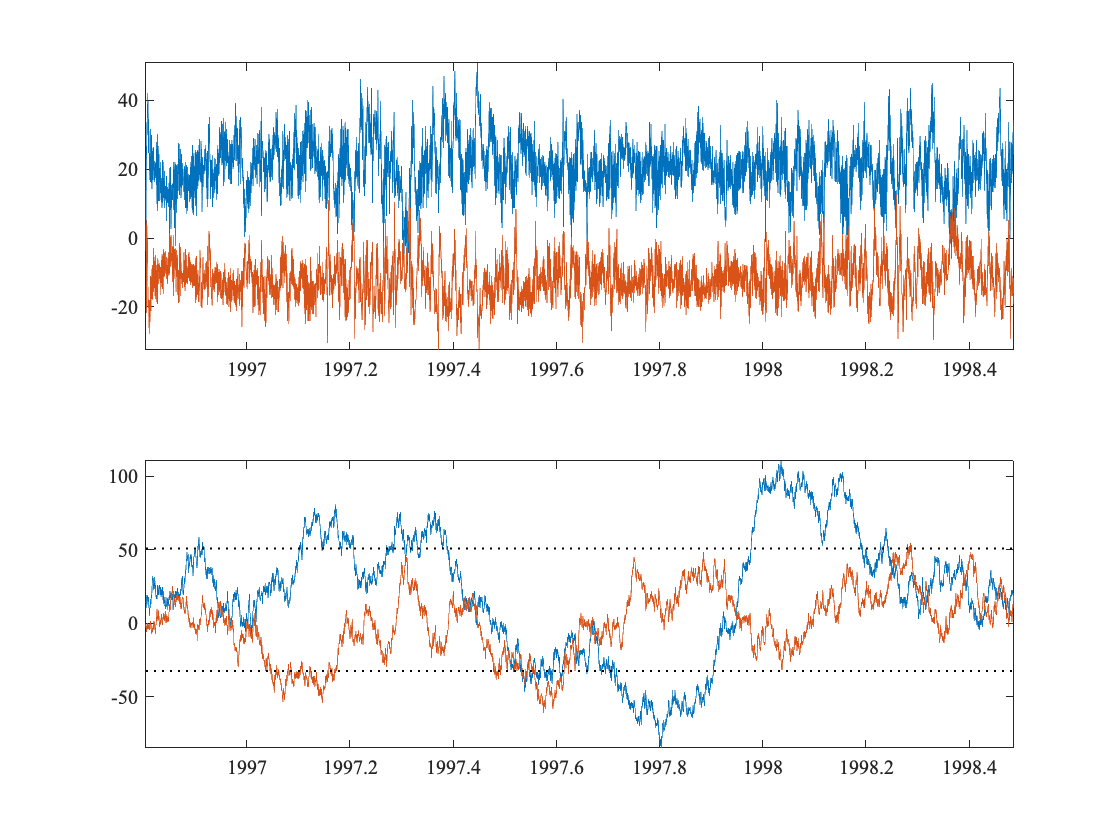I hope you see the pattern here. White noise matches the low frequencies, while red noise matches the high frequencies; we can't match both with the same process. Actually, this type of behavior turns out to be quite common. For this reason, myself and colleagues have spent time investigating a hybrid process that transitions from white noise to red noise at a specified intermediate frequency.
At this point we will introduce some new terminology. Red noise with a spectrum ofis also known as Brownian motion, with a subtle distinction. The distinction is whether the process is defined only at discrete points, as has been done here by cumsumming disrete white noise, or is instead defined for continuous time t.
Strictly speaking, Brownian motion isnoise that is defined on continuous time, not discrete time. In fact, if we have a continuous-time process with a spectral slope of -2, and we sample it at discrete times and take the spectrum, the spectrum will have more energy at high frequencies that the -2 slope would predict. Why? Because of aliasing. Spectral contributions from unresolved, higher frequencies are wrapped into the resolved spectrum. Therefore it is not the same to have anspectrum in discrete or continuous time.
Nevertheless, this is in some sense a relatively minor detail, and it is fair to say that our cumsummed white noise is an approximation to Brownian motion.

# Damped Fractional Brownian Motion

This hybrid process is called the Matérn process, after the first person who proposed it. As we showed in the above paper, it is also the same as damped fractional Brownian motion. The term fractional Brownian motion refers to a generalization of Brownian motion in which the spectral slope can take on a range of values, not just -2; in fact it can be anywhere from -1 to -3. Fractional Brownian motion was introduced by Mandelbrot (of fractal fame) and Van Ness in 1968.
The damping term refers to a modification of fractional Brownian motion such that instead of going off to infinitely large values near zero frequecy, as implied by an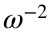slope for example, the value of the spectrum becomes a constant. As we show in our paper, this is precisely what is required if you would like a velocity spectrum to correspond to finite diffusivities, as one does in Lagrangian drifter applications, because the zero-frequency value of the velocity spectrum is exactly the diffusivity. Because Brownian motion can be thought of as the motion of a particle subject to random perturations, the damping term has the literal interpretation of a friction acting to damp out those motions. This modification also removes the problem that prevents fractional Brownian motion from being well-defined for slopes steeper than -3, and so the Matérn process can have slopes or -1 or anything larger.
In jLab, damped fractional Brownian motion is generated by the function maternoise, which I think you will find easy to use. The arguments to maternoise are described in the comments.
dt=2/24; %The sampling interval
sigma=std(cv);%The desired standard deviation
alpha=1; %One-half of the desired spectral slope
lambda=0.6; %The damping parameter, or desired frequency, in *units of* 1/dt
cveps=maternoise(dt,N,sigma,alpha,lambda,'fast');
%The 'fast' specifies the use of an algorithm which greatly
%speeds up the generation, so you should use this.
[f,sppeps,snneps]=mspec(2/24,cveps,psi);
clf,
plot(f/2/pi,[snn spp snneps sppeps]),xlim([f(2) f(end)]/2/pi),xlog,ylog,ylim(10.^[-1 3])
linestyle T U D k
title('Rotary Spectra of Signal vs. Noise')
vlines(lambda/2/pi,'k:')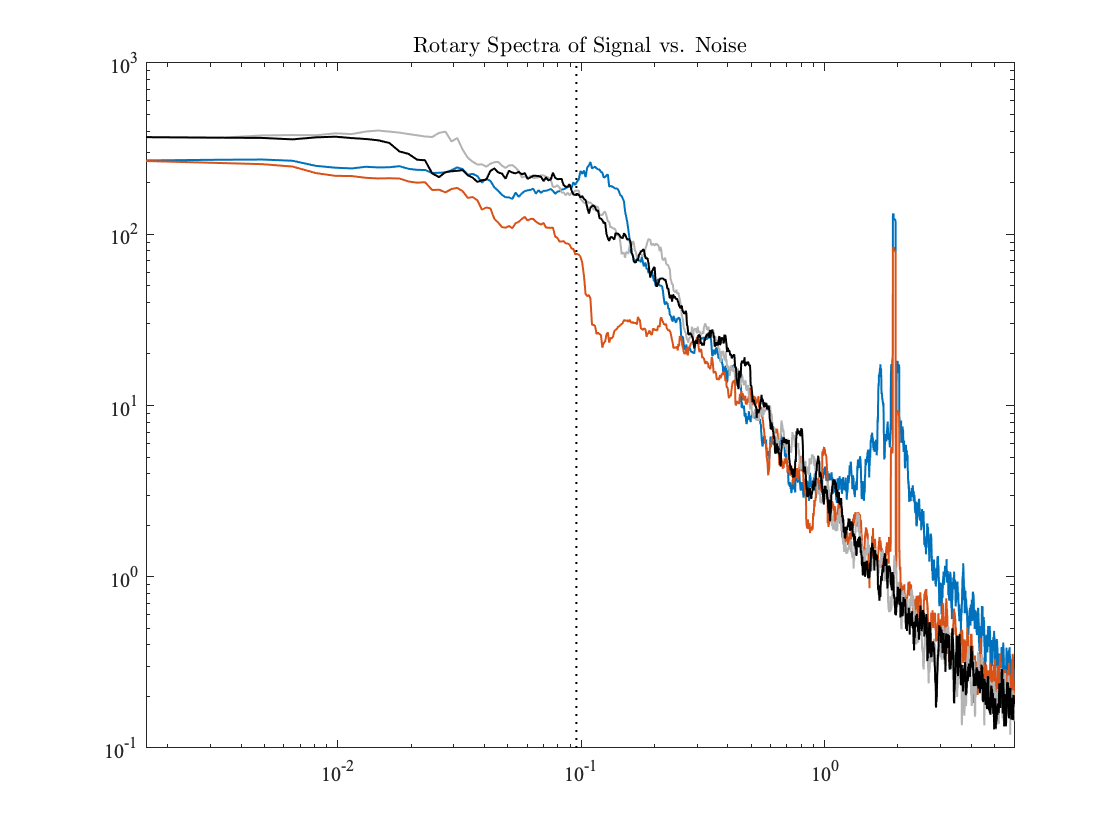This call to generates a random process that has standard deviation σ, high-frequency spectral slope of 2α, and which transitions to a white spectrum around frequency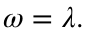That's all there is to it. So to make use of this process, all you need to do is to choose these three parameters to match your data. As you can see, the choices we have made provide an excellent fit to the observed spectrum from our current meter mooring, apart from (i) the inertial band at negative frequencies, (ii) the narrow semidiurnal tidal peak, and (iii) the low-frequency peak/valley at around one cycle per day.
The spectrum of the Matérn process has the simple formwhere Ais an amplitude setting the spectral slope. As shown in our paper, Ais related to the standard deviation by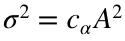/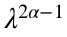, where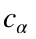is a known function of the slope parameter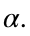Observe that at high frequencies, this becomes a power-law spectrum with a slope of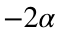, while at low frequencies it becomes white.
Let's take a look also at the time series.
figure,
subplot(2,1,1),uvplot(yearfrac(num),cv),axis tight,ax=axis;
subplot(2,1,2),uvplot(yearfrac(num),cveps+mean(real(cv))),axis tight
hlines(ax(3:4),'k:') %The lines show the y-axis of the upper plot